# Probability with Dice

Probability with Dice• The sample space is the list of all possible outcomes.
• A dice has 6 sides which are all equally likely to be rolled.
• Each side has a different number written on it.
• We can roll a: 1, 2, 3, 4, 5 or a 6.
• We say that the sample space for a dice is: 1, 2, 3, 4, 5, 6.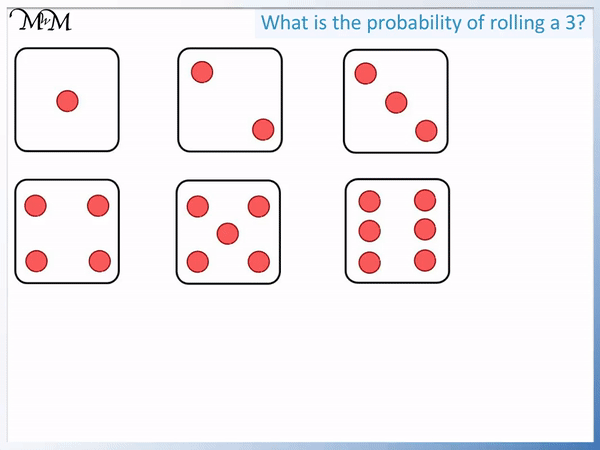• Probability is a measure of the chance of something happening.
• We can write the probability of rolling a 3 as a fraction.
• There are 6 different outcomes in total that we can roll.
• So the fraction is out of 6.
• Only one side of the dice is a ‘3’.
• So the probability of rolling a three is   1 / 3   .
• The number three makes up 1 out of 6 sides of the dice and on average will be rolled once every six rolls.

A dice has six equally likely outcomes: 1, 2, 3, 4, 5 and 6.

The probability of rolling each number is 1 out of 6.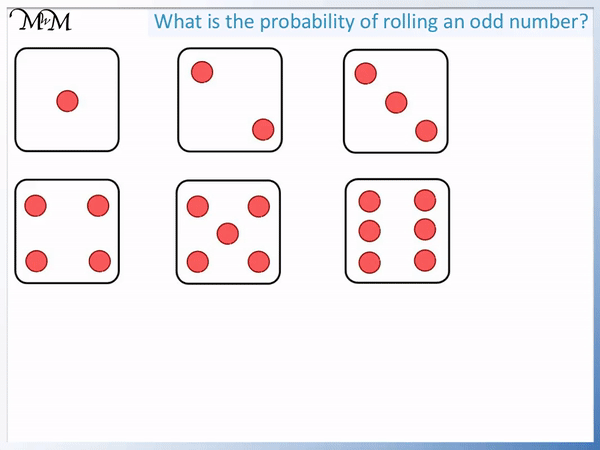• We will write the probability of rolling an odd number on a dice as a fraction.
• The odd numbers are 1, 3 and 5.
• This is 3 of the 6 sides of the dice.
• The probability of rolling an odd number on a dice is   3 / 6  .
•   3 / 6   is the same as   1 / 2
• You can expect an odd number to be rolled half of the time.# Probability with Dice

Probability measures the chance of something happening. Each different result is known as an outcome.In this lesson we are looking at probability with rolling dice. We will figure out the probabilities of different outcomes that can occur when rolling a dice.

The list of all possible different outcomes is known as the sample space.The sample space for rolling a dice is shown below.The sample space for a dice is 1, 2, 3, 4, 5, 6.

A dice contains six sides, which each have an equal chance of occurring when the dice is rolled.In the example below we are asked, “What is the probability of rolling a 1?”.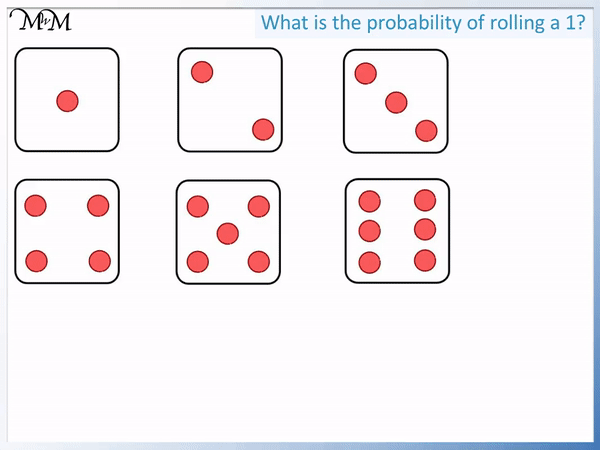We know that there are 6 possible outcomes in total:

1, 2, 3, 4, 5 or 6

There is only one face of the dice with a ‘1’ on it.

The probability of rolling a one is   1 / 6  .

There is only one face out of six faces that have a ‘1’ on it.

Here is another example.

“What is the probability of rolling a 3 on a dice?”There is only one face that has a ‘3’.

1 out of 6 sides of the dice contain a ‘3’.

The probability of rolling a 3 is   1 / 6  .

We can see that the probability of rolling any single number from 1 to 6 on a dice is   1 / 6  .

In the example below we are asked, “What is the probability of rolling a 2 or a 5?”.Looking at our dice sample space, we still have 6 total outcomes: 1, 2, 3, 4, 5 or 6.

We can see that ‘2’ and ‘5’ are two of these faces on the dice.

The probability of rolling a 2 or a 5 is   2 / 6.

Two of the six faces are outcomes that we want.

In our sample space list we can see this:

1, 2, 3, 4, 5, 6

2 / 6   can be simplified to 1 / 3  by halving both the numerator and denominator of the fraction.

This means that on average, a 2 or 5 will appear on a dice every three times that the dice is rolled.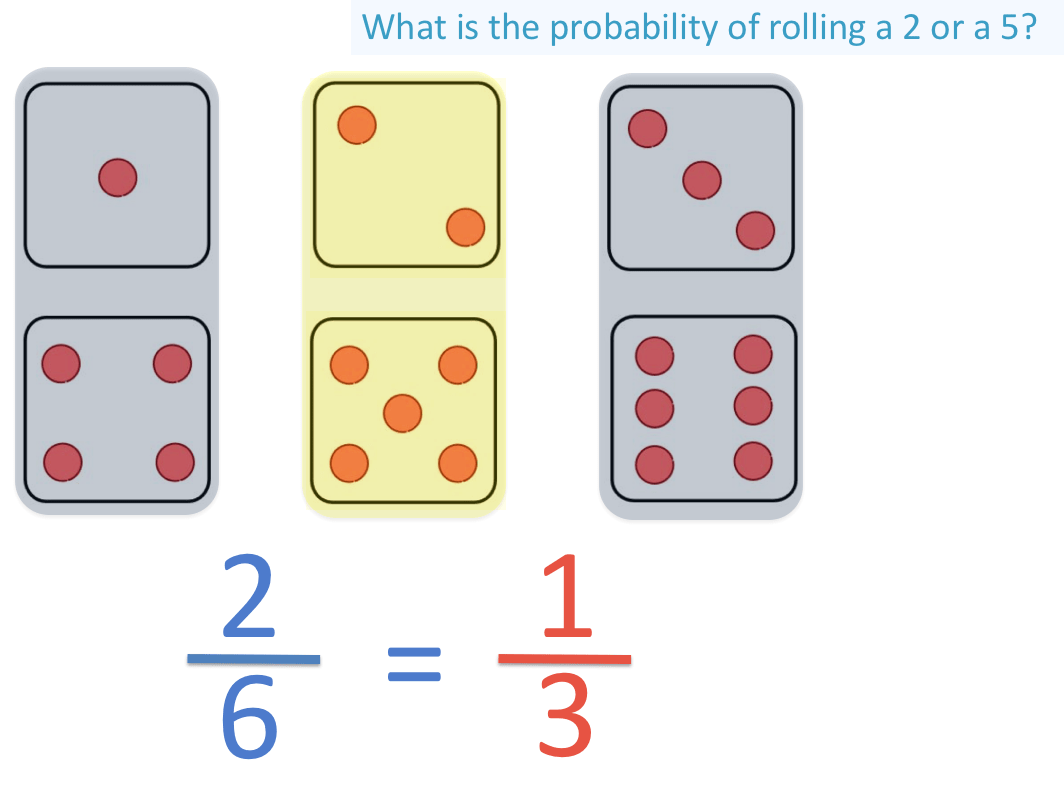Here is another example of dice probability.

“What is the probability of rolling a number greater than 4?”.The numbers greater than 4 are: ‘5’ and ‘6’.

This is 2 out of 6 possible outcomes and so the probability is   2 / 6   .

Again this probability can be simplified to   1 / 3 .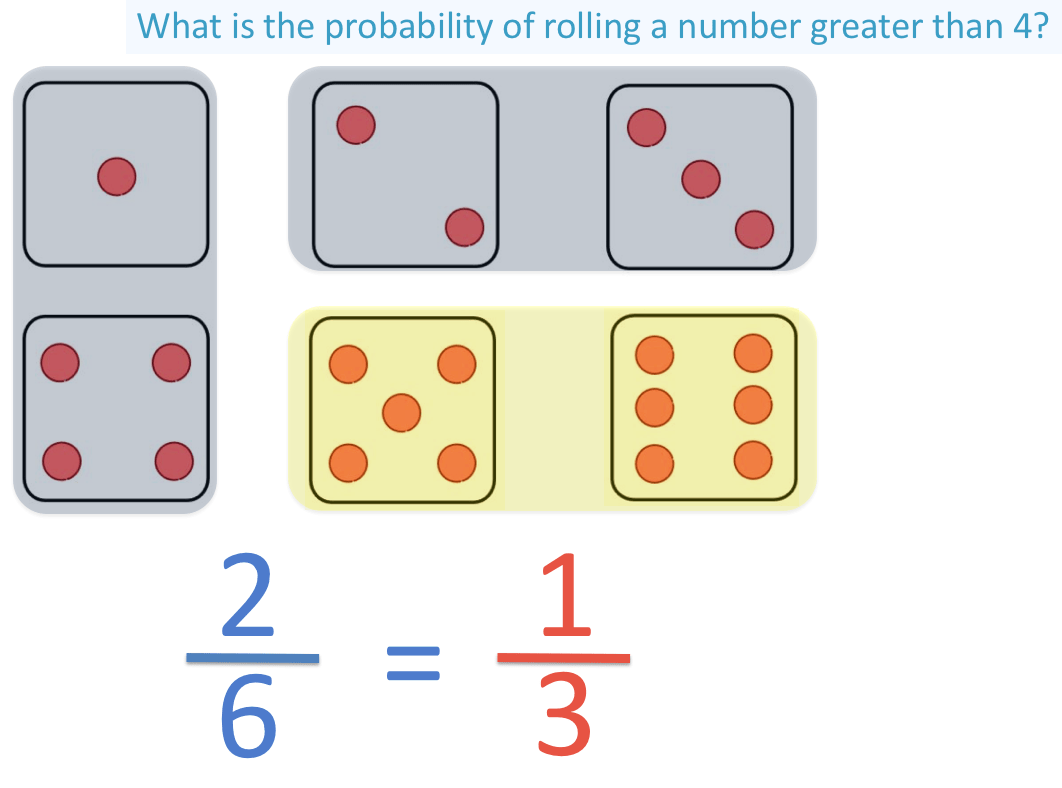It is important to be careful not to include the number ‘4 in this example.

4 is not greater than 4 and so, we do not include it.

‘5’ and ‘6’ are the only numbers on a dice that are greater than 4.

Here is another example.

“What is the probability of rolling a 3 or less?”.

In this example, we have the number 3 or the numbers less than 3.

So we have ‘3’ and the numbers ‘2’ and ‘1’.

This is different from the previous example because we are allowed to include 3 and the numbers below it.

The word or gives this away.This is 3 out of the six possible outcomes.

On our sample space list we can see this as:

1, 2, 3, 4, 5, 6

The probability of rolling a 3 or less on a dice is   3 / 6.

3 is half of 6 and so   3 / 6 can be simplified to   1 / 2.

When rolling a dice we can expect to roll a ‘3 or less’ half of the time.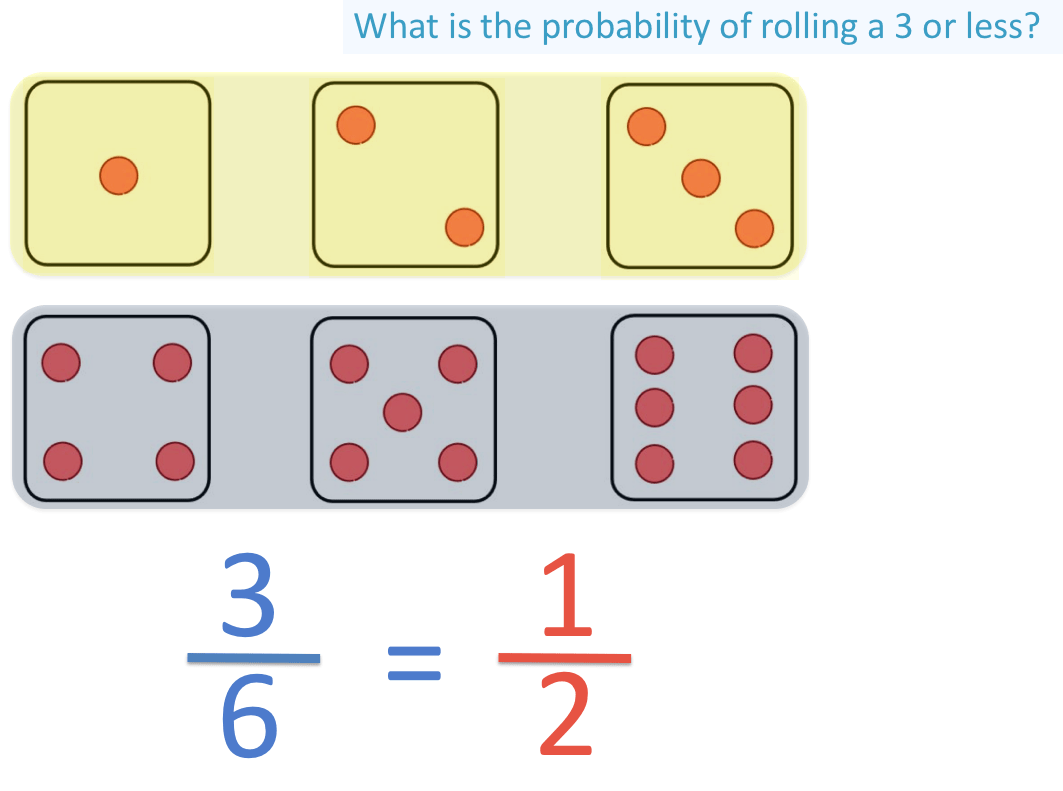In the example below we are asked, “What is the probability of rolling an odd number?”.The odd numbers on a dice are: 1, 3 and 5

This is 3 out of the 6 numbers.

The probability is   3 / 6 .

This probability can be simplified to   1 / 2.Half of the numbers on a regular die are odd.

The opposite of rolling an odd number is to roll an even number.There are 3 out of 6 outcomes on a dice that are even: 2, 4 and 6.

And so, the probability of rolling an even number on a dice is   3 / 6 .

We could have figured this probability out using out last example.

If   3 / 6 numbers on the dice are odd, then the remaining numbers are even.

3 / 6 and   3 / 6 add to make   6 / 6, which is all 6 faces of our dice.

When we roll our dice, we expect an odd number half of the time and an even number the other half of the time.Now try our lesson on Drawing and Interpreting Pictograms (Pictographs) where we learn how to draw pictograms to represent data.